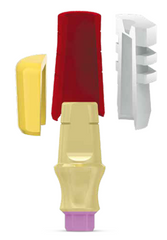MIS

# Conical CPK (Complete Prosthetic Kit)

CK-NPK41
• Sale
• Regular price

The Complete Prosthetic Kit (CPK) is a comprehensive set designed for the full restoration of parallel inserted implants and restoration of a single implant case. The CPK includes all required components for the fabrication of cement retained restorations: an abutment, impression cap, comfort cap, casting sleeves and an analog.Cat. No. Platforms Implant Dims. (mm) CK-NPK41 NP C1 Ø4, H=1, C=4 VK-NPK41 NP V3 Ø4, H=1, C=4 CK-CPK41 SP V3/C1 Ø4.8, H=1, C=4 CK-NPK42 NP C1 Ø4, H=2, C=4 VK-NPK42 NP V3 Ø4, H=2, C=4 CK-CPK42 SP V3/C1 Ø4.8, H=2, C=4 CK-NPK43 NP C1 Ø4, H=3, C=4 VK-NPK43 NP V3 Ø4, H=3, C=4 CK-CPK43 SP V3/C1 Ø4.8, H=3, C=4 CK-NPK44 NP C1 Ø4, H=3, C=4 VK-NPK44 NP V3 Ø4, H=3, C=4 CK-CPK44 SP V3/C1 Ø4.8, H=3, C=4 CK-NPK61 NP C1 Ø4, H=1, C=6 VK-NPK61 NP V3 Ø4, H=1, C=6 CK-CPK61 SP V3/C1 Ø4.8, H=1, C=6 VK-CPK61 SP V3/C1 Ø5.5, H=1, C=6 CK-WPK61 WP C1 Ø5.5, H=1, C=6 CK-NPK62 NP C1 Ø4, H=2, C=6 VK-NPK62 NP V3 Ø4, H=2, C=6 CK-CPK62 SP V3/C1 Ø4.8, H=2, C=6 VK-CPK62 SP V3/C1 Ø5.5, H=2, C=6 CK-WPK62 WP C1 Ø5.5, H=2, C=6 CK-NPK63 NP C1 Ø4, H=3, C=6 VK-NPK63 NP V3 Ø4, H=3, C=6 CK-CPK63 SP V3/C1 Ø4.8, H=3, C=6 VK-CPK63 SP V3/C1 Ø5.5, H=3, C=6 CK-WPK63 WP C1 Ø5.5, H=3, C=6 CK-NPK64 NP C1 Ø4, H=4, C=6 VK-NPK64 NP V3 Ø4, H=4, C=6 CK-CPK64 SP V3/C1 Ø4.8, H=4, C=6 VK-CPK64 SP V3/C1 Ø5.5, H=4, C=6 CK-WPK64 WP C1 Ø5.5, H=4, C=6
Material: Titanium